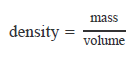# Problem: The density of iron is 7.87 g/mL. What is the mass of a 4.00 cm × 4.00 cm × 1.00 cm block of iron?Express your answer to three significant figures and include the appropriate units.By definition,Typical units of density are g/mL or g/cm3.1 mL = 1 cm3

###### FREE Expert Solution
86% (422 ratings)View Complete Written Solution
###### Problem Details

The density of iron is 7.87 g/mL. What is the mass of a 4.00 cm × 4.00 cm × 1.00 cm block of iron?

Express your answer to three significant figures and include the appropriate units.

By definition,Typical units of density are g/mL or g/cm3.

1 mL = 1 cm3

Frequently Asked Questions

What scientific concept do you need to know in order to solve this problem?

Our tutors have indicated that to solve this problem you will need to apply the Density of Geometric Objects concept. You can view video lessons to learn Density of Geometric Objects. Or if you need more Density of Geometric Objects practice, you can also practice Density of Geometric Objects practice problems.